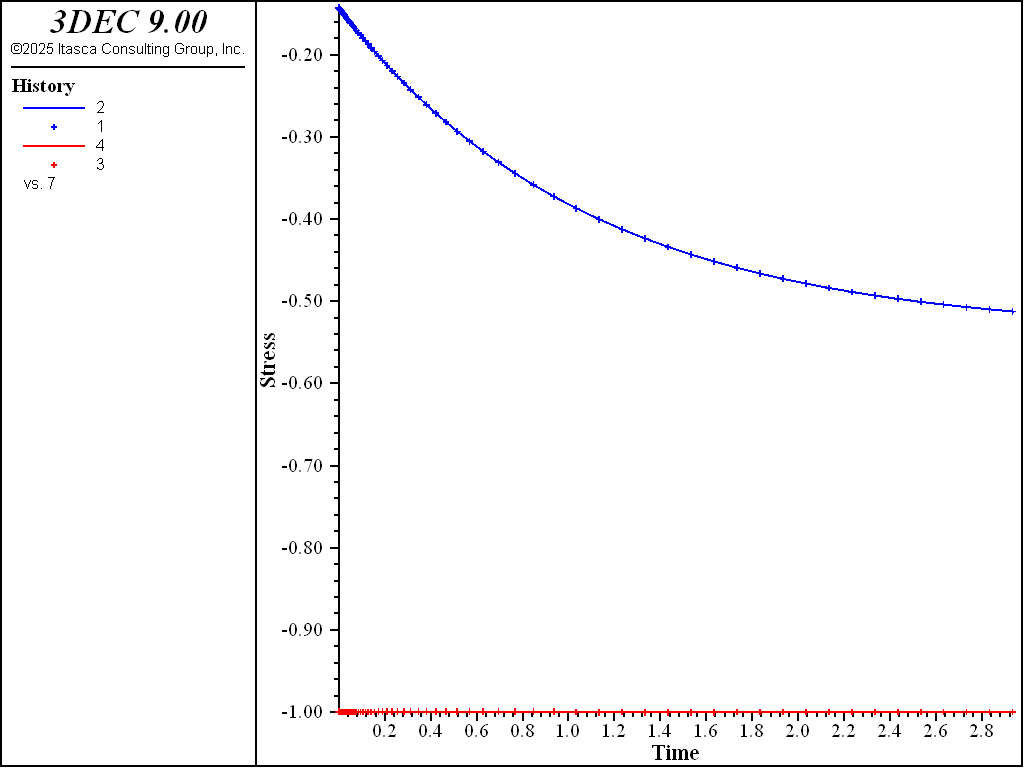# Kelvin Model: Oedometer Test

Note

To view this project in 3DEC, use the menu command Help ► Examples…. Choose “CreepMaterialModels/ OedometerKelvin” and select “OedometerKelvin.prj” to load. The project’s main data files are shown at the end of this example.

This example compares the numerical and analytical solutions of an oedometer test carried out on a generalized Kelvin substance (Kelvin cell in series with a shear spring). In the oedometer test, the base of the sample is fixed, lateral deformations are prevented, and a constant vertical load $$P$$ is applied at the top of the specimen.

The analytical solution for vertical strain and stresses is

(1)$\epsilon_{zz}=- {{P} \over {K}} b \left[ c \left( 1 - e^{-at} \right) + 1 \right]$
(2)$\sigma_{zz} = -P$
(3)$\sigma_{xx} = \sigma_{yy} = {{K} \over {2}} \left( 3 \epsilon_{zz} + {{P} \over {K}} \right)$

where $$b$$, $$a$$, and $$c$$ are the three constants:

(4)$b = {{K} \over {K + 4G/3}}$
(5)$a = {{G_{K}/G + b} \over {\eta / G}}$
(6)$c = {{1 - b} \over {G_{k} / G + b}}$

$$K$$, $$G_{K}$$, $$G$$ are bulk and shear moduli of the substance ($$G_{k}$$ is for the Kelvin cell, $$G$$ is for the spring in series), and $$\eta$$ is the viscosity.

The numerical simulation is carried out simultaneously on two samples, each represented by one zone, using the Burgers model and the Burgers-Mohr model.

Because no value is assigned to the property viscosity-maxwell, the Maxwell dashpot is not taken into account by the models. Also, the cohesion property is set to a high value to prevent triggering of the plasticity logic for the Burgers-Mohr model. The initial state is first obtained by cycling the model to elastic equilibrium. Velocities are then reset to zero. For the viscous response, the initial timestep is set to a small value ($$\Delta t$$ = 10-6) compared to the ratio of viscosity over shear modulus ($$\eta /G_K$$ = 1.0). With the choice of creep timestep parameter settings used in the example, the timestep increases by a factor of 1.01 when the out-of-balance force ratio is less than 10-4, until $$\Delta t$$ = 0.1. At the end of the simulation, the response is for two shear moduli, $$G_{k}$$ and $$G$$, acting in series (long-term behavior). Figure 1 and Figure 2 show the agreement between analytical solutions and numerical predictions for stresses and strains in the two samples.Figure 1: Oedometer test on a Kelvin substance: analytical and numerical stress values versus time.Figure 2: Oedometer test on a Kelvin substance: analytical and numerical strain values versus time.

Data Files

OedometerKelvin.dat

;------------------------------------------------------------
;       Oedometer test -- KElvin substance
;-------------------------------------------------------------------
model new
fish automatic-create off
model title "Oedometer test --- 'Kelvin' substance"
model configure creep
model large-strain off

fish define ini_cons
global c_bu = 2.
global c_sh = 2.
global c_ksh = 1.
global c_kvi = 2.
global c_pr = -1.
local a   = 3. * (c_bu + 4. * c_sh / 3.) / (2. * c_sh)
local al  = c_ksh + 3. * c_bu / (2. * a)
global c_a = al / c_kvi
global c_b = 3. * c_pr / (a * a * al)
global c_c =  c_pr / (c_bu + 4. * c_sh / 3.)
global c_d = 3. * c_bu
end
[ini_cons]

; --- model ---
block create brick 0 1
block zone generate edgelength 1.0
block zone cmodel assign burgers-mohr
block zone property density 1 bulk [c_bu] shear-maxwell [c_sh] shear-kelvin [c_ksh] viscosity-kelvin [c_kvi]
block zone property cohesion 1E20 tension 1E20
block face apply stress 0.0 0.0 [c_pr] 0 0 0 range position-z 1
block gridpoint apply velocity-x 0 range position-x 0 1
block gridpoint apply velocity-y 0 range position-y 0 1
block gridpoint apply velocity-z 0 range position-z 0

; --- elastic equilibrium ---
model solve

; --- histories ---
program call 'analytical.fis'
fish history sxx
fish history ana_sxx
fish history szz
fish history ana_szz
block history displacement-z position 0.0 0.0 1.0
fish history ana_ezz
model history creep time-total
model history timestep

; --- reset velocities to zero ---
block gridpoint initialize velocity (0,0,0)
; --- viscous behaviour ---
model creep timestep starting 1.e-6
model creep timestep minimum  1.e-6
model creep timestep maximum  1.e-2
model solve time-total=3.
model save 'kelvin'
program return